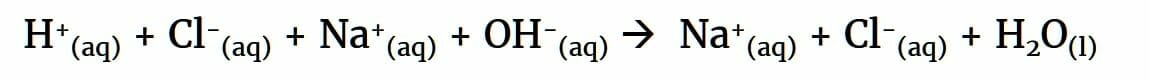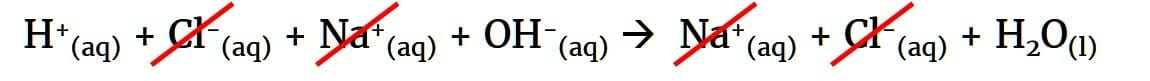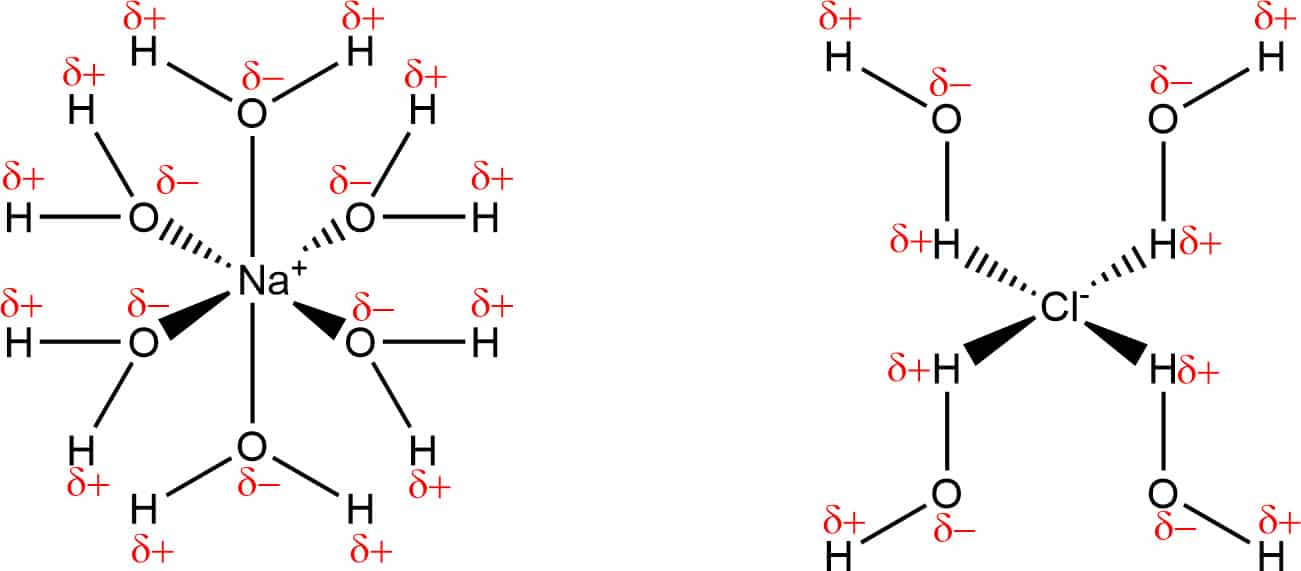# Step-by-step guide to understanding and writing ionic equations

Aimed at GCSE, A Level and IB students, this guide will help you master the difficult skills of writing half-equations and ionic half-equations

## What are ionic equations?

Ionic equations are chemical equations that show only ions that participate in a chemical reaction. In other words, the ions that react together in solution and form new substances. The other ions that don’t participate are called spectator ions.

The purpose of ionic equations is they simplify chemical equations by eliminating the species that aren’t undergoing any change. This is very useful, particularly when dealing with redox reactions which are complicated enough without spectator ions getting in the way.

In the neutralisation reaction between dilute hydrochloric acid and sodium hydroxide solution, a salt and water are formed. We can write the balanced equation for this:To simplify this, the first step is to split up the compounds into ions. The result is the ionic equation:Now, we can see that we have like terms on each side of the equation in the form of Na+ and Cl ions. As you would do in maths, these can be cancelled – they appear on both sides unchanged, hence they are not doing anything in the reaction and can be removed from it. These are the spectator ions.The result – which is often called the net ionic equation – shows that in this neutralisation reaction, all that really happens is a H+ ion reacts with a OH ion to form water.Na+ and Cl don’t play any role in the reaction whatsoever.

The interesting thing (and useful fact for revision purposes) is that all neutralisation reactions have this same ionic equation. You can swap Na+ for any cation and Cl for any anion and the ionic equation for neutralisation will still be H+ + OH –> H2O

## Which substances ionise in ionic equations?

When students first learn how to write ionic equations, the part they find challenging is identifying which substances in the equation will ionise, or form ions. It’s helpful to first think about what this means.

For reactions occurring in water, ionisation (also known as dissociation) means a substance has been split up into ions by the action of water. The diagram below helps to visualise this process. In the case of an ionic compound, the strong forces of attraction between the oppositely-charged ions get broken down by water molecules and each ion becomes surrounded by water molecules:Above GCSE level, you’ll learn that the ions have become solvated, meaning water has formed coordinate bonds to the ions:Substances that readily ionise in water, and therefore easily dissolve in it, include:

• Ionic compounds (those formed from metals and non-metals)
• Salts (substances where the H+ ion in an acid has been replaced by a metal or NH4+ ion)
• Acids
• Alkalis (soluble bases)

These groups represent an enormous number of compounds, many of which would be unfamiliar to students. For example, we can quite easily identify a familiar substance like NaCl as an ionic compound that ionises to form Na+ and Cl in solution, but what about something like K2S4O6?

Fortunately, we have a state symbol and a set of rules to help determine which substances do and don’t ionise.

## The state symbol (aq), aqueous

A substance being split up by the action of water means it has formed a solution in water – it has dissolved. This we show using the state symbol aq, meaning the substance has formed an aqueous solution. With very few exceptions, a substance in aqueous solution will be ionised, because the process of dissolving usually requires ionisation.

If a substance has the state symbol aqueous in a chemical equation, it means that the substance is soluble and will usually be ionised.

The exception to this is when (aq) means a substance has dissolved without ionising.

An example of this would be Cl2(aq), or chlorine water, which you wouldn’t show ionising to form Cl+ and Cl for instance. (Note that Cl2 in water does form ions, but it’s through reaction with water and not what we’re considering here).

## The solubility rules

If the chemical equation doesn’t include state symbols, or if it’s an aqueous substance but you’re not 100% certain that it’s soluble, you can use the solubility rules:

SubstanceSolubility
Common salts of sodium, potassium and ammoniumAll soluble
NitratesAll soluble
SulfatesSoluble, except lead, barium and calcium
CarbonatesInsoluble, except sodium, potassium and ammonium
HydroxidesInsoluble, except sodium, potassium, barium and ammonium
OxidesInsoluble, except sodium, potassium and ammonium

Anything that this table indicates is soluble can be ionised in a chemical reaction.

## Don’t ionise solids, liquids or gases!

Lastly, don’t ionise solids or gases present as reactants or products in chemical reactions, and don’t ionise liquids unless it’s a molten ionic compound.

This is especially important for precipitation reactions, where solutions are mixed and an insoluble solid forms. When that happens, don’t ionise the precipitate that has the state symbol (s) – it is formed as a precipitate because it’s not soluble and so won’t break up to form ions.

You should also not ionise water in ionic equations. Water does ionise in solution, forming H+ and OH ions, but as you’ll discover post-GCSE, its ionisation is negligible compared to other ionic substances.

## Don’t ionise polyatomic ions!

Whilst sulfuric acid (H2SO4) ionises to form sulfate ions (SO42-), you can’t ionise sulfate ions any further. Similarly, you wouldn’t ionise nitrate ions (NO3), carbonate ions (CO32-), phosphate ions (PO43-) and ions like chlorate(I) (ClO) and iodate(V) (IO3). These polyatomic ions are stable in solution compared to the ions you’d form if they broke up further.

The table below shows some examples of substances that can and can’t be ionised.

SubstanceDoes it ionise?
HNO3(aq)Yes, forming H+ and NO3
H2S(aq)Yes, forming 2H+ and S2-
SO42-(aq)No (you won’t get S6+ and 4O2-)
NaOH(aq)Yes, forming Na+ and OH
CuS(aq)Yes, forming Cu2+ and S2-
AgCl(s)No – it’s a solid
BaSO4(s)No – it’s a solid
Cu(OH)2(s)No – it’s a solid
Al2O3(l)Yes, forming Al3+ and O2-
Cl2(aq), Br2(aq) and I2(aq)No

## Steps for writing ionic equations

With the theory out of the way, learn these steps to write the ionic equation for any chemical reaction:

1. Write the balanced symbol equation
2. Identify the soluble compounds using the solubility rules or the aqueous state symbol
3. Split up the soluble compounds into ions
4. Cancel ions that appear on both sides of the equation
5. Write out the resulting ionic equation

Let’s see how this works for a reaction that is described in words (interpreting this and turning it into a balanced symbol equation is an important skill that can be tested in the exams):

When aluminium metal is added to iron(III) nitrate solution, the pale yellow colour disappears leaving a colourless solution containing metallic particles.

Hopefully you can recognise this as a metal displacement reaction in which the more reactive metal aluminium displaces the less reactive metal iron from its compounds.

Write the balanced symbol equation

Al(s) + Fe(NO3)3(aq)  → Al(NO3)3(aq) + Fe(s)

Identify the soluble compounds using the solubility rules or the aqueous state symbol

Fe(NO3)3 is a nitrate, so will be soluble, as will Al(NO3)3. Both of these salts will dissociate into ions. The metals will not be soluble.

Split up the soluble compounds into ions

Al(s) + Fe3+(aq) + 3NO3(aq)  → Al3+(aq) + 3NO3(aq) + Fe(s)

Cancel ions that appear on both sides of the equation

Al(s) + Fe3+(aq) + 3NO3(aq)  → Al3+(aq) + 3NO3(aq) + Fe(s)

Write out the resulting ionic equation

Al(s) + Fe3+(aq)  → Al3+(aq) + Fe(s)

## What are ionic half-equations?

A close relative of ionic equations, ionic half-equations are exclusively used for redox reactions. The name redox is derived from the fact that two reactions occur simultaneously, a reduction and an oxidation.

Redox reactions are therefore two-part reactions, and it’s possible to split the overall chemical equation representing a redox reaction into two chemical equations, one of the reduction, one for the oxidation. These are known as half-equations. The two half-equations combined give the overall equation. Ionic half-equation simply refers to the fact that we simplify the half-equation by only showing the ions that undergo change. Spectators ions are left out.

## Steps for writing ionic half-equations

1. Write the balanced symbol equation
2. Identify the soluble compounds using the solubility rules or the aqueous state symbol
3. Split up the soluble compounds into ions
4. Cancel ions that appear on both sides of the equation
5. Write out the resulting ionic equation
6. Write a half-equation for the oxidation and reduction reaction, balancing charges with electrons

Example

We can use another metal displacement reaction to illustrate how ionic half-equations are written. Magnesium is a more reactive metal than lead, so will displace lead from its compounds. In this example, magnesium is added to a solution of lead(II) nitrate:

Mg(s) + Pb(NO3)2(aq) → Mg(NO3)2(aq) + Pb(s)

The first steps of the procedure are the same as for writing ionic equations. First of all, split the soluble aqueous compounds into ions:

Mg(s) + Pb2+(aq) + 2NO3(aq) → Mg2+(aq) + 2NO3(aq) + Pb(s)

Then cancel spectator ions:

Mg(s) + Pb2+(aq) + 2NO3(aq) → Mg2+(aq) + 2NO3(aq) + Pb(s)

Write out the resulting ionic equation:

Mg(s) + Pb2+(aq) → Mg2+(aq) + Pb(s)

Now, write the half-equation for the reduction reaction. Lead changes oxidation state from +2 to 0, so it is the element getting reduced. Since reduction is gain, the electrons go on the LHS:

2e + Pb2+(aq) → Pb(s)

Next, write the half-equation for the oxidation reaction. Magnesium changes oxidation state from  0 to +2, so it is the element getting oxidised. Since oxidation is loss, the electrons go on the RHS:

Mg(s) → Mg2+(aq) + 2e

To summarise:

• The overall reaction is: Mg(s) + Pb(NO3)2(aq) → Mg(NO3)2(aq) + Pb(s)
• The ionic equation is: Mg(s) + Pb2+(aq) → Mg2+(aq) + Pb(s)
• The reduction half-equation is: 2e + Pb2+(aq) → Pb(s)
• The oxidation half-equation is: Mg(s) → Mg2+(aq) + 2e

## Practise worksheets

Worksheet on writing ionic equations

Answer to worksheet on writing ionic equations